# Chapter 7: Multi-Dimensional Arrays## Overview

Static multi-dimensional arrays and their representation. Row-major storage format and the access formula. Passing multi-dimensional arrays as function arguments. Dynamic multi-dimensional arrays.

It is natural and practical to extend the notion of one-dimensional arrays - that is, arrays accessible via a single index - to multi-dimensional arrays accessible through multiple indexes. We will illustrate the concepts on two-dimensional arrays, since the principles and issues are the same as for higher-dimensional arrays yet the notation and visualization are much simpler.

Consider a simple definition of a static two-dimensional int array x , where the first index ranges from 0 to 2 and the second index ranges from 0 to 3. For a better illustration, let us fill the array with some distinctive values using the C/C++ initialization:

` int x = {0,1,2,3,10,11,12,13,20,21,22,23}; `

A typical visualization of such an array is a table, as depicted in Figure 7.1.Figure 7.1: Visualization of the two-dimensional array x

Since memory is the focal interest of this book, the first question the reader must ask concerns the "dimension" of the visualization: the memory is dimensionless (or at best it is a one-dimensional array of bytes), so how is it that we can visualize the array x as a two-dimensional table? The second question ought to be: Why is the first index represented as rows of the table and the second index as columns ? Moreover, is this really an accurate representation? The following simple program will "visualize" the array in both the row/column and the column/row manner.

` int main()    {       int x = {0,1,2,3,10,11,12,13,20,21,22,23};       int i, j;       for(i = 0; i < 3; i++) {        for(j = 0; j < 4; j++)         printf("%2d ",x[i][j]);        putchar('\n');       }       putchar('\n');       for(j = 0; j < 4; j++) {        for(i = 0; i < 3; i++)         printf("%2d ",x[i][j]);        putchar('\n');       }    return 0;    } `

producing output

` 0  1  2  3    10 11 12 13    20 21 22 23     0 10 20     1 11 21     2 12 22     3 13 23 `

The answer to both questions is simple. The visualization has nothing to do with the physical dimensions of the storage. We are quite used to this; for example, a two-dimensional fax image is sent across one-dimensional phone wires. It is simply a convention to visualize two-dimensional arrays in this particular way. You could just as well visualize them in the column/row fashion (the second part of the program's output) and everything would be satisfactory, as long as you consistently worked with the same visualization. Hereafter, we shall work with the standard row/column visualization of two-dimensional arrays.

Now we can examine how a two-dimensional array is really stored in memory. The C/C++ language uses the row-major format, in which the array is (like the one-dimensional static array case discussed in Chapter 6) a contiguous statically allocated memory segment and the "rows" of the array are stored there one after another, as shown in Figure 7.2. And just as in the one-dimensional case, a single pointer suffices to hold the base address.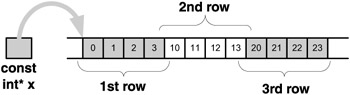Figure 7.2: The row-major storing format of a two-dimensional array x

Access to the array items is performed according to the row-major access formula, which transforms a reference x[i][j] to an indirection expression *(x+i*n+j) , where n is the row size of x . For example: x is translated to *(x+0*4+0)=*x , hence the item with the value 0 is being accessed; and x is translated to *(x+1*4+2)=*(x+6) , hence the item with the value 12 is being accessed.

The reader should note a subtle difference between the indexing mechanism of one-dimensional arrays and that used for multi-dimensional arrays. For one-dimensional arrays, the indexing really is the indirection and so x[i]=*(x+i) , whereas for multi-dimensional arrays the indexing is only based on the indirection with some additional information needed and so x[i][j]=*(x+i*n+j) (where n is the additional information in this example). Hence, we cannot simply view a two-dimensional array as a pointer with a "body" as we did for the one-dimensional case, even though Figure 7.2 does not look that much different from Figure 6.3. The compiler represents the two-dimensional array x as depicted in Figure 7.2 and " knows " the row size of x (which happens to be 4) from the definition of x ; thus it can translate any expression x[i][j] to *(x+i*4+j) . Throughout the compilation, the compiler simply must treat the pointer int* x differently from "regular" int* pointers - unlike the one-dimensional case, for which the compiler treats int* x like any other pointer.

We have just illustrated that a multi-dimensional array is accessed at run time as a one-dimensional array, for the stored version of the array really is one-dimensional. The multiple indexes are transformed to proper single indexes via the row-major access formula. Thus, as in the one-dimensional case, in the multi-dimensional case the pointer representing the array is passed by value ; this allows direct access to the actual array locally from within the function, as if the array were passed by reference. In order to facilitate access to array items through indexing, the compiler must have additional information (in the two-dimensional case, the row size) in order to translate the indexing to a proper indirection.

It is essential for the compiler to know the row size, but how does it obtain this value? While parsing the array's definition, the compiler extracts the row size - for instance, in int x , the last index limit (4) is the size it needs to know. However, in the previous chapter we learned that when declaring a one-dimensional array (most often as a function argument) we need not bother to specify the size. Can we do the same for multi-dimensional arrays? Clearly not, for the compiler must have this additional information in order to do its job. Thus, we may leave out the size of the first index but cannot leave out the size of any other index. We may therefore declare x either as x or as x[] .

We briefly consider the three-dimensional array int x . The row-major format will store the items in the following order (we list only the indexes):

` 000,001,010,011,020,021,030,031,100,101,110,111,120,121,130,       131,200,201,210,211,220,221,230,231 `

The first eight entries constitute a two-dimensional array x  with the first index fixed at 0 (i.e., x [i][j]=x[i][j] ), consisting of four rows of size 2: {000,001} , {010,011} , {020,021} , {030,031} . The middle eight entries constitute a two-dimensional array x 1  with the first index fixed at 1, consisting of four rows of size 2: {100,101} , {110,111} , {120,121} , {130,131} . Finally, the last eight entries constitute a two-dimensional array x 2  with the first index fixed at 2, also consisting of four rows of size 2: {200,201} , {210,211} , {220,221} , {230,231} . The row-major formula comes to x[i][j][k]=*(x+i*8+j*2+k) .

In general, row-major storage is a recursive format that stores an n- dimensional array x[i 1 ]..[i n ] as a contiguous sequence of L 1 arrays that are ( n -1)-dimensional: x [i 2 ]..[i n ] , x 1 [i 2 ]..[i n ] ,...,and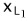[i 2 ]..[i n ] , where x k [i 2 ]..[i n ] denotes the array x with the first index fixed as k and where L 1 denotes the limit of the first index. The row-major formula in its generality is given by

` x[i  1  ][i  2  ]..[i   n   ]=*(x+i  1  *L  2  *..*L   n   + i  2  *L  3  *..*L   n   +..                                  + i   n-    2  *L   n-    1  *L   n   +i   n-    1  *L   n   + i   n   ) `

where L i denotes the size (limit) of the i th index, i =2,..., n.

The standard visualization of a three-dimensional array is a cube, where the first index is interpreted as the height (see Figure 7.3), the second index as the width (Figure 7.4), and the third index as the depth (Figure 7.5).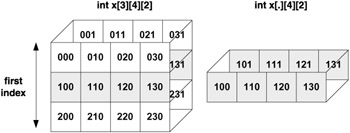Figure 7.3: The "slice" when the first index is fixed as 1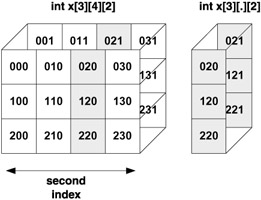Figure 7.4: The "slice" when the second index is fixed as 2Figure 7.5: The "slice" when the third index is fixed as 2

Even though it is simple and efficient, the treatment of multi-dimensional arrays as if they were one-dimensional poses some problems for the dynamic version. In the one-dimensional case we need only provide a "body" for a pointer and, voil  , we have an array. With multi-dimensional arrays we cannot do this, for the compiler cannot treat a pointer p as an array and translate p[i][j] to *(p+i*n+j) . Not only is the compiler unaware of what n can possibly be - even the programmer might not know. We must therefore rely on the automatic indexing as indirection for the one-dimensional case. Let us illustrate this on int** p . We have p[i][j]=*(p[i]+j)=*(*(p+i)+j) and this does make sense, since int** p , so *(p+i) is of the type int* and hence *(*(p+i)+j) is of the type int . We thus require a two-stage solution, for p+i must point somewhere, and application of the indirection operator *(p+i) gets the address stored there; hence *(p+i)+j points somewhere, and another application of the indirection operator *(*(p+i)+j) retrieves the number stored there. Here is a code to create a dynamic two-dimensional int array p :

` int** p; p = malloc(3*sizeof(int*)); if (p == NULL) error(); for(i = 0; i < 3; i++)  p[i]= malloc(4*sizeof(int)); /* let us put in values as sample accessing of the array items */ a=0; for(i = 0; i < 3; i++)  for(j = 0; j < 4; j++)   p[i][j] = a++; `

Figure 7.6 illustrates the dynamic two-dimensional array p created in this sample program.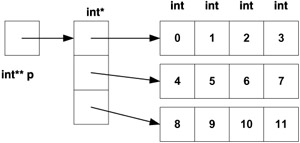Figure 7.6: Dynamically created two-dimensional array p

Note that we have actually created four one-dimensional arrays. One is an array of pointers; let us call this the row array, as each item of this array is a pointer to a one-dimensional int array representing a row. The pointer p that represents the whole array points to the row array. In a sense we have created a dynamic one-dimensional array of dynamic one-dimensional arrays. For a three-dimensional dynamic array we would do exactly the same and create a one-dimensional dynamic array of two-dimensional dynamic arrays, and similarly for higher dimensions.

Let us now investigate exactly what (say) p refers to in our previous sample code. The automatic indexing through indirection of p refers to the pointer to the middle row, and hence the automatic indexing through indirection of p refers the second item of the middle row (with value 5). Thus the structure exhibits the correct kind of behavior with respect to indexing.

It is interesting that - despite the high cost of their creation - dynamic multi-dimensional arrays may facilitate faster execution in comparison to static arrays. Access to a static array must always be calculated using the row-major formula and hence employs multiplication, an expensive operation. Yet access to a dynamic array is just a simple series of dereferencing, which is much less expensive. Thus, for a program with a large number of accesses to the array, the dynamic version may improve over-all performance. For a program running in a system with constrained memory size and needing a large multi-dimensional array, it may be advantageous for the dynamic version of the array to consist of many unrelated smaller segments rather than a single large one as the static version requires. However, there are also potential drawbacks: a dynamic multi-dimensional array that spans a wide portion of the address space may complicate and slow down caching and/or paging and thus degrade performance.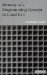Memory as a Programming Concept in C and C++
ISBN: 0521520436
EAN: 2147483647
Year: 2003
Pages: 64

Similar book on Amazon

flylib.com © 2008-2017.
If you may any questions please contact us: flylib@qtcs.net